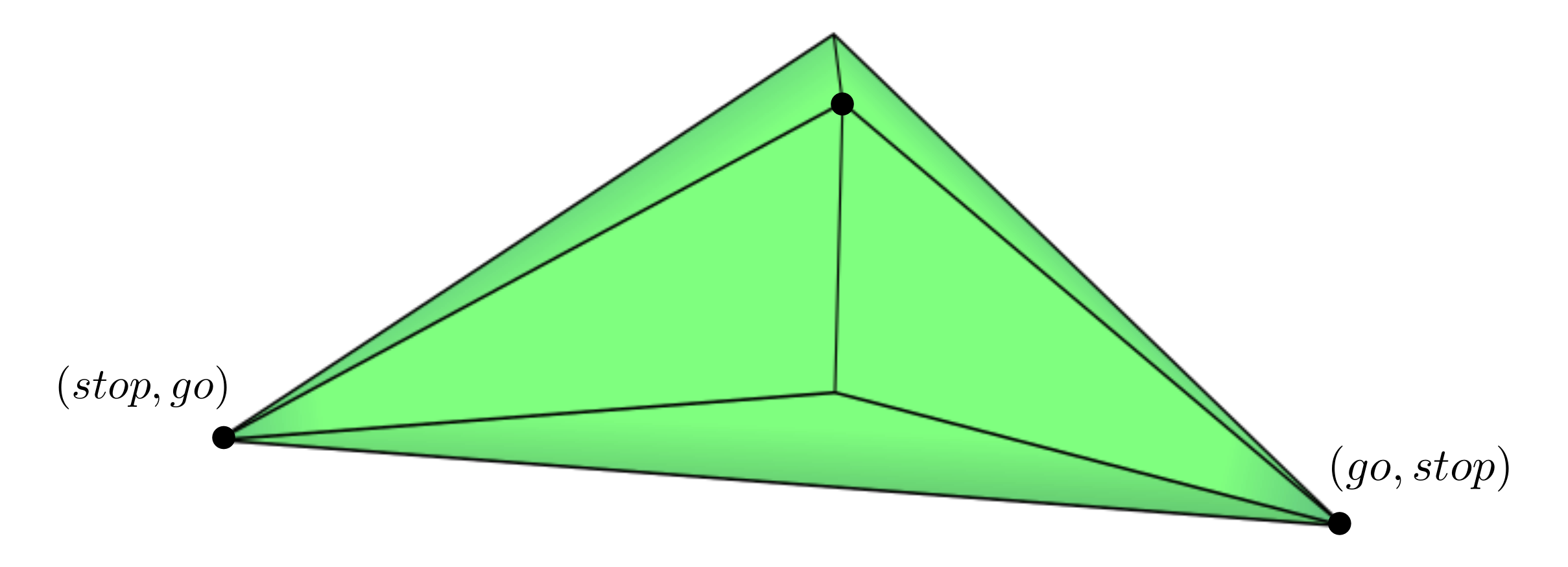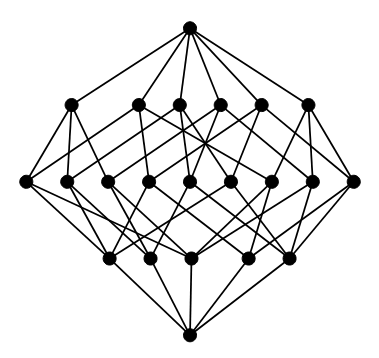# Combinatorics of Correlated Equilibria¶

Marie-Charlotte Brandenburg, Benjamin Hollering, and Irem Portakal: Combinatorics of Correlated Equilibria.Abstract: We study the correlated equilibrium polytope $$P_G$$ of a game $$G$$ from a combinatorial viewpoint. We introduce the region of full-dimensionality for this class of polytopes, and prove that it is a semialgebraic set for any game. Through the use of the oriented matroid strata, we propose a structured method for describing combinatorial types of $$P_G$$, and show that for $$(2 \times n)$$-games, the algebraic boundary of each stratum is the union of coordinate hyperplanes and binomial hypersurfaces. We provide a computational proof that for $$(2 \times 3)$$-games there exists a unique combinatorial type of maximal dimension.

## 3d Model¶

The set of correlated equilibria of a fixed game is a polytope, and the Nash equilibria of the game are the intersection of this polytope with the Segre variety inside the probability simplex. Below is a 3d model of the correlated equilibrium polytope (blue) inside the probability simplex (yellow) the Segre variety (orange), and the Nash equilibria (green). Click here to see the 3d model in full screen: [Full Screen]

## Supplementary Material¶

This page contains supplementary material for the computations performed in the article. All files with ending .nb are run with $$\verb|Mathematica|$$. Files endig with .py are run in $$\verb|SageMath|$$ and diles endig with .m2 are run in $$\verb|Macaulay2|$$. A file with ending .ipnynb is a Jupyter Notebook that is executed with a $$\verb|SageMath|$$ kernel.

### Computing the Polytope from Payoffs¶

We provide code to compute the correlated equilbrium polytope and the correlated equilibrium cone of a fixed $$(d_1 \times \dots \times d_n)$$-game with payoff tensor in $$X$$ in $$\verb|SageMath|$$. First, download the file correlated_polytope_cone.py and place it in the same folder as your Jupyter Notebook for SageMath. An example of a $$(2 \times 3)$$-game can then be computed by the lines

from correlated_polytope_cone import *
D_i = [2,3]
X = [
[9,4],     # X_{11} = [X_{11}^(1), X_{11}^(2)]
[4,0],     # X_{12} = [X_{12}^(1), X_{12}^(2)]
[6,2],     # X_{13} = [X_{13}^(1), X_{13}^(2)]
[5,5],     # X_{21} = [X_{21}^(1), X_{21}^(2)]
[3,8],     # X_{22} = [X_{22}^(1), X_{22}^(2)]
[9,7]      # X_{23} = [X_{23}^(1), X_{23}^(2)]
]
P = correlated_equilibrium_polytope(D_i, X, base_ring = QQ)
C = correlated_equilibrium_cone(D_i, X, base_ring = QQ)


The input $$D\_i = [d_1,\dots,d_n]$$ is the type of the game, e.g. D_i = [2,3] for a $$(2 \times 3)$$-game as in the example above. X above corresponds to the payoff table.

 Player 2 $$s_1^{(2)}$$ $$s_2^{(2)}$$ $$s_3^{(2)}$$ Player 1 $$s_1^{(1)}$$ (9,4) (4,0) (6,2) $$s_2^{(1)}$$ (5,5) (3,8) (9,7)

### Section 5: Combinatorial Types of Correlated Equilibrium Polytopes¶

#### Code for Random Sampling Combinatorial Types of Polytopes¶

Credits
Project page created: 09/14/2022
Project contributors: Marie-Charlotte Brandenburg, Benjamin Hollering, and Irem Portakal
Software used: SageMath (Version 9.6), Mathematica (Version 13.0), Macaulay2 (Version 1.20)
System setup used: MacBook Pro with macOS Monterey 12.5.1, Processor 3,1 GHz Quad-Core Intel Core i7, Memory 16 GB 2133 MHz LPDDR3, Graphics Intel HD Graphics 630 1536 MB.
Corresponding authors of this page: Marie-Charlotte Brandenburg (marie.brandenburg@mis.mpg.de) and Benjamin Hollering (benjamin.hollering@mis.mpg.de)